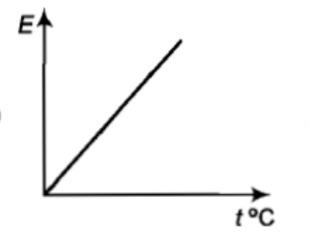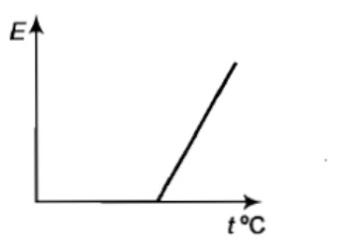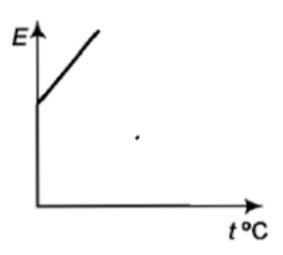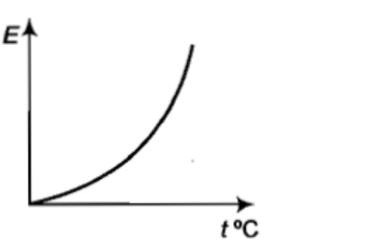An isolated system-

1. is a specified region where transfer of energy and mass takes place

2. is a region of constant mass and only energy is allowed through the closed boundaries

3. is one in which mass within the system is not necessarily constant.

4. cannot transfer either energy or mass to or from the surroundings.

Subtopic:  Ideal Gas Equation |
To view explanation, please take trial in the course below.
NEET 2022 - Target Batch - Aryan Raj Singh
To view explanation, please take trial in the course below.
NEET 2022 - Target Batch - Aryan Raj Singh

Difficulty Level:

The pressure and temperature of two different gases are P and T having the volume V for each.  They are mixed keeping the same volume and temperature, the pressure of the mixture will be-                                                          [Pb. PMT 1997, 98; DPMT 1999; MH CET 2003]

1. P/2

2. P

3. 2P

4. 4P

Subtopic:  Ideal Gas Equation |
To view explanation, please take trial in the course below.
NEET 2022 - Target Batch - Aryan Raj Singh
To view explanation, please take trial in the course below.
NEET 2022 - Target Batch - Aryan Raj Singh

Difficulty Level:

If the mean free path of atoms is doubled then the pressure of the gas will become:

1. P/4

2. P/2

3. P/8

4. P

Subtopic:  Mean Free Path |
To view explanation, please take trial in the course below.
NEET 2022 - Target Batch - Aryan Raj Singh
To view explanation, please take trial in the course below.
NEET 2022 - Target Batch - Aryan Raj Singh

Difficulty Level:

A diatomic molecule has how many degrees of freedom-

(1) 3            (2) 4           (3) 5              (4) 6

Subtopic:  Law of Equipartition of Energy |
To view explanation, please take trial in the course below.
NEET 2022 - Target Batch - Aryan Raj Singh
To view explanation, please take trial in the course below.
NEET 2022 - Target Batch - Aryan Raj Singh

Difficulty Level:

A thermally insulated piston divides a container into two compartments.  Volume, temperature, and pressure in the right compartment are 2V, T, and 2P, while in the left compartment the respective values are V, T, and P.  If the piston can slide freely, then in the final equilibrium position, the volume of the right-hand compartment will be-
1. V
2. $\frac{3V}{5}$
3. $\frac{9V}{4}$
4. $\frac{12V}{5}$

Subtopic:  Ideal Gas Equation |
To view explanation, please take trial in the course below.
NEET 2022 - Target Batch - Aryan Raj Singh
To view explanation, please take trial in the course below.
NEET 2022 - Target Batch - Aryan Raj Singh

Difficulty Level:

The graph which represents the variation of mean kinetic energy of molecules with temperature $t°C$ is ?

(1)(2)(3)(4)Subtopic:  Kinetic Energy of an Ideal Gas |
To view explanation, please take trial in the course below.
NEET 2022 - Target Batch - Aryan Raj Singh
To view explanation, please take trial in the course below.
NEET 2022 - Target Batch - Aryan Raj Singh

Difficulty Level:

${V}_{rms},{V}_{av},{V}_{mp}$ are root mean square, average and most probable speeds of molecules of a gas obeying Maxwellian velocity distribution. Which of the following statements is correct?

1. ${V}_{rms}<{V}_{av}<{V}_{mp}$

2. ${V}_{rms}>{V}_{av}>{V}_{mp}$

3.

4. ${V}_{avg}>{V}_{rms}<{V}_{mp}$

Subtopic:  Types of Velocities |
To view explanation, please take trial in the course below.
NEET 2022 - Target Batch - Aryan Raj Singh
To view explanation, please take trial in the course below.
NEET 2022 - Target Batch - Aryan Raj Singh

Difficulty Level:

Gas is found to obey the law ${P}^{2}V$ = constant. The initial temperature and volume are ${T}_{0}$ and ${V}_{0}$. If the gas expands to a volume $3{V}_{0}$, its final temperature becomes:

1. $\frac{{T}_{0}}{3}$

2. $\frac{{T}_{0}}{\sqrt{3}}$

3. $3{T}_{0}$

4. None of these

Subtopic:  Ideal Gas Equation |
To view explanation, please take trial in the course below.
NEET 2022 - Target Batch - Aryan Raj Singh

Difficulty Level:

For a gas, the r.m.s speed at 800 K is:

1. Four times the value at 200 K

2. Half the value at 200 K

3. Twice the value at 200 K

4. Same as at 200 K

Subtopic:  Types of Velocities |
To view explanation, please take trial in the course below.
NEET 2022 - Target Batch - Aryan Raj Singh
To view explanation, please take trial in the course below.
NEET 2022 - Target Batch - Aryan Raj Singh

Difficulty Level:

The average kinetic energy of a helium atom at $30°C$ is:                                                                                           [MP PMT 2004]

1. Less than 1 eV

2. A few KeV

3. 50-60 eV

4. 13.6 eV

Subtopic:  Kinetic Energy of an Ideal Gas |
To view explanation, please take trial in the course below.
NEET 2022 - Target Batch - Aryan Raj Singh
To view explanation, please take trial in the course below.
NEET 2022 - Target Batch - Aryan Raj Singh

Difficulty Level: